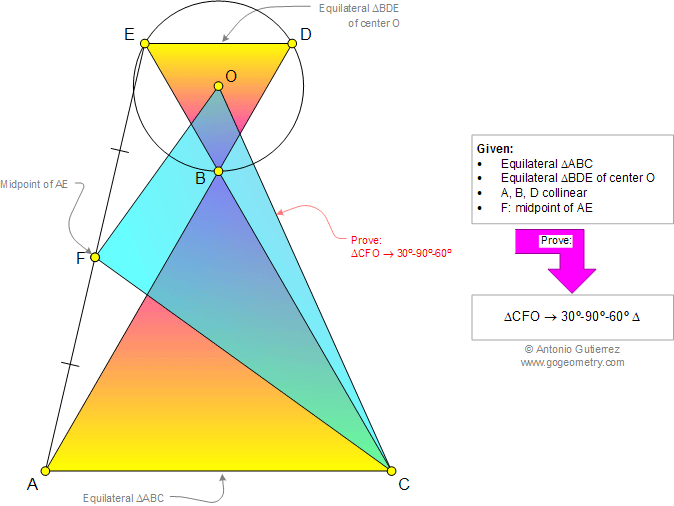# Geometry Problem 956: Two Equilateral Triangles, Center, Collinear Points, Midpoint, Right Triangle, 30, 60, 90 Degrees. Level: School, College, Mathematics Education

< PREVIOUS PROBLEM  |  NEXT PROBLEM >

 The figure shows an equilateral triangle ABC and equilateral triangle BDE of center O such that A, B, and D are collinear. If F is the midpoint of AE, prove that the triangle CFO is a 30-90-60 degrees triangle.Home | Search | Geometry | Problems | All Problems | Open Problems | Visual Index | 10 Problems | Problems Art Gallery |  Art | 951-960 | Triangles | Equilateral Triangle | Collinear Points | Midpoint | Angles 30, 60, 120 degrees | Right Triangle 30-60 | by Antonio Gutierrez